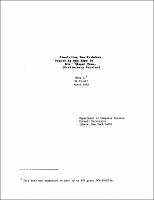## Simulating Two Pushdown Stores by One Tape in $O(n^{1.5}\sqrt{logn}$) Time. (Preliminary Version)##### Abstract
Based on two graph separator theorems, one old (the Lipton-Tarjan planar separator theorem) and one new, we present two unexpected upper bounds and resolve several open problems for on-line computations: (1) 1 tape nondeterministic machines can simulate 2 pushdown stores in time $O(n^{1.5} \sqrt{logn})$ (true for both on-line and off-line machines). Together with the $\Omega (n^{1.5} \sqrt{logn})$ lower bound by the author [L1], this solved the open problem 1 in [DGPR] for the 1 tape vs. 2 pushdown case. It also disproves the commonly conjectured $\Omega (n^{2})$ lower bound. (Note, the $\Omega (n^{2})$ lower bound has been proved for the deterministic case [M, L, V].) (2) the languages defined by [M] and [F], aimed to obtain optimal lower bound for 1 tape nondeterministic machines, can be accepted in $O(n^{2}loglogn / \sqrt{logn})$ and $O(n^{1.5}\sqrt{logn})$ time by a 1 tape TM, respectively. (3) 3 pushdown stores are better than 2 pushdown stores. This answers open problem 3 of [DG]. (An $\Omega(n^{4/3} / log^{e} n)$ lower bound is also obtained.) (4) 1 tape can nondeterministically simulate 1 queue in $O(n^{1.5} \sqrt{logn})$ time. (The lower bounds were: $\Omega(n^{2})$ for deterministic case [V] and $\Omega(n^{4/3} / logn)$ [L1] for nondeterministic case.)
1985-03
##### Publisher
Cornell University
##### Keywords
computer science; technical report
##### Previously Published As
http://techreports.library.cornell.edu:8081/Dienst/UI/1.0/Display/cul.cs/TR85-667
technical report